# 2.2 Flow around a cylinder

Tutorial path:

In this example we shall investigate potential flow around a cylinder using thepotentialFoam solver. This example introduces the following OpenFOAM features:

• non-orthogonal meshes;
• generating an analytical solution to a problem in OpenFOAM;
• use of a dynamic code to generate the block vertices;
• use of a coded function object to compare results against the analytical solution.

### 2.2.1 Problem specification

The problem is defined as follows:

Solution domain
The domain is 2 dimensional and consists of a square domain with a cylinder collocated with the centre of the square as shown in Figure 2.15.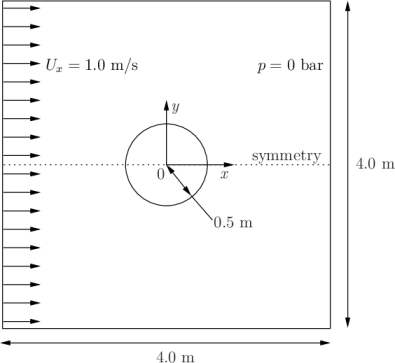Figure 2.15: Geometry of flow round a cylinder

Governing equations

• Mass continuity for an incompressible fluid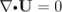(2.14)

• Pressure equation for an incompressible, irrotational fluid assuming steady-state conditions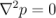(2.15)

Boundary conditions

• Inlet (left) with fixed velocity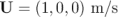.
• Outlet (right) with a fixed pressure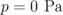.
• No-slip wall (bottom);
• Symmetry plane (top).
Initial conditions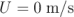,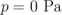— required in OpenFOAM input files but not necessary for the solution since the problem is steady-state.
Solver name
potentialFoam: a potential flow code, i.e. assumes the flow is incompressible, steady, irrotational, inviscid and it ignores gravity.
Case name
cylinder case located in the \$FOAM_TUTORIALS/basic/potentialFoam directory.

### 2.2.2 Note on potentialFoam

potentialFoam is a useful solver to validate OpenFOAM since the assumptions of potential flow are such that an analytical solution exists for cases whose geometries are relatively simple. In this example of flow around a cylinder an analytical solution exists with which we can compare our numerical solution. potentialFoam can also be run more like a utility to provide a (reasonably) conservative initial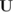field for a problem. When running certain cases, this can useful for avoiding instabilities due to the initial field being unstable. In short, potentialFoam creates a conservative field from a non-conservative initial field supplied by the user.

### 2.2.3 Mesh generation

Mesh generation using blockMesh has been described in tutorials in the User Guide. In this case, the mesh consists of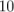blocks as shown in Figure 2.16.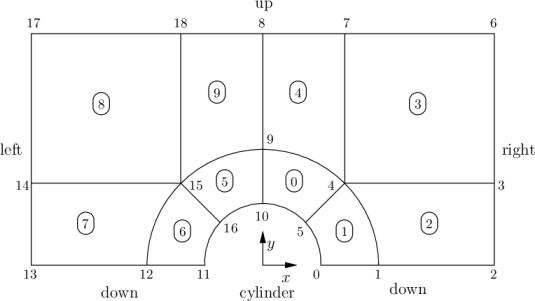Figure 2.16: Blocks in cylinder geometry

Remember that all meshes are treated as 3 dimensional in OpenFOAM. If we wish to solve a 2 dimensional problem, we must describe a 3 dimensional mesh that is only one cell thick in the third direction that is not solved. In Figure 2.16 we show only the back plane of the geometry, along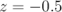, in which the vertex numbers are numbered 0-18. The other 19 vertices in the front plane,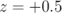, are numbered in the same order as the back plane, as shown in the mesh description file below:

1/*--------------------------------*- C++ -*----------------------------------*\
2| =========                 |                                                 |
3| \\      /  F ield         | OpenFOAM: The Open Source CFD Toolbox           |
4|  \\    /   O peration     | Version:  v2006                                 |
5|   \\  /    A nd           | Website:  www.openfoam.com                      |
6|    \\/     M anipulation  |                                                 |
7\*---------------------------------------------------------------------------*/
8FoamFile
9{
10    version     2.0;
11    format      ascii;
12    class       dictionary;
13    object      blockMeshDict;
14}
15// * * * * * * * * * * * * * * * * * * * * * * * * * * * * * * * * * * * * * //
16
17scale   1;
18
19vertices #codeStream
20{
21    codeInclude
22    #{
23        #include "pointField.H"
24    #};
25
26    code
27    #{
28        pointField points(19);
29        points  = point(0.5, 0, -0.5);
30        points  = point(1, 0, -0.5);
31        points  = point(2, 0, -0.5);
32        points  = point(2, 0.707107, -0.5);
33        points  = point(0.707107, 0.707107, -0.5);
34        points  = point(0.353553, 0.353553, -0.5);
35        points  = point(2, 2, -0.5);
36        points  = point(0.707107, 2, -0.5);
37        points  = point(0, 2, -0.5);
38        points  = point(0, 1, -0.5);
39        points = point(0, 0.5, -0.5);
40        points = point(-0.5, 0, -0.5);
41        points = point(-1, 0, -0.5);
42        points = point(-2, 0, -0.5);
43        points = point(-2, 0.707107, -0.5);
44        points = point(-0.707107, 0.707107, -0.5);
45        points = point(-0.353553, 0.353553, -0.5);
46        points = point(-2, 2, -0.5);
47        points = point(-0.707107, 2, -0.5);
48
49        // Duplicate z points
50        label sz = points.size();
51        points.setSize(2*sz);
52        for (label i = 0; i < sz; i++)
53        {
54            const point& pt = points[i];
55            points[i+sz] = point(pt.x(), pt.y(), -pt.z());
56        }
57
58        os  << points;
59    #};
60};
61
62
63blocks
64(
65    hex (5 4 9 10 24 23 28 29) (10 10 1) simpleGrading (1 1 1)
66    hex (0 1 4 5 19 20 23 24) (10 10 1) simpleGrading (1 1 1)
67    hex (1 2 3 4 20 21 22 23) (20 10 1) simpleGrading (1 1 1)
68    hex (4 3 6 7 23 22 25 26) (20 20 1) simpleGrading (1 1 1)
69    hex (9 4 7 8 28 23 26 27) (10 20 1) simpleGrading (1 1 1)
70    hex (15 16 10 9 34 35 29 28) (10 10 1) simpleGrading (1 1 1)
71    hex (12 11 16 15 31 30 35 34) (10 10 1) simpleGrading (1 1 1)
72    hex (13 12 15 14 32 31 34 33) (20 10 1) simpleGrading (1 1 1)
73    hex (14 15 18 17 33 34 37 36) (20 20 1) simpleGrading (1 1 1)
74    hex (15 9 8 18 34 28 27 37) (10 20 1) simpleGrading (1 1 1)
75);
76
77edges
78(
79    arc 0 5 (0.469846 0.17101 -0.5)
80    arc 5 10 (0.17101 0.469846 -0.5)
81    arc 1 4 (0.939693 0.34202 -0.5)
82    arc 4 9 (0.34202 0.939693 -0.5)
83    arc 19 24 (0.469846 0.17101 0.5)
84    arc 24 29 (0.17101 0.469846 0.5)
85    arc 20 23 (0.939693 0.34202 0.5)
86    arc 23 28 (0.34202 0.939693 0.5)
87    arc 11 16 (-0.469846 0.17101 -0.5)
88    arc 16 10 (-0.17101 0.469846 -0.5)
89    arc 12 15 (-0.939693 0.34202 -0.5)
90    arc 15 9 (-0.34202 0.939693 -0.5)
91    arc 30 35 (-0.469846 0.17101 0.5)
92    arc 35 29 (-0.17101 0.469846 0.5)
93    arc 31 34 (-0.939693 0.34202 0.5)
94    arc 34 28 (-0.34202 0.939693 0.5)
95);
96
97boundary
98(
99    down
100    {
101        type symmetryPlane;
102        faces
103        (
104            (0 1 20 19)
105            (1 2 21 20)
106            (12 11 30 31)
107            (13 12 31 32)
108        );
109    }
110    right
111    {
112        type patch;
113        faces
114        (
115            (2 3 22 21)
116            (3 6 25 22)
117        );
118    }
119    up
120    {
121        type symmetryPlane;
122        faces
123        (
124            (7 8 27 26)
125            (6 7 26 25)
126            (8 18 37 27)
127            (18 17 36 37)
128        );
129    }
130    left
131    {
132        type patch;
133        faces
134        (
135            (14 13 32 33)
136            (17 14 33 36)
137        );
138    }
139    cylinder
140    {
141        type symmetry;
142        faces
143        (
144            (10 5 24 29)
145            (5 0 19 24)
146            (16 10 29 35)
147            (11 16 35 30)
148        );
149    }
150);
151
152mergePatchPairs
153(
154);
155
156// ************************************************************************* //

### 2.2.4 Boundary conditions and initial fields

Edit the case files to set the boundary conditions in accordance with the problem description in Figure 2.15, i.e. the left boundary should be an Inlet, the right boundary should be an Outlet and the down and cylinder boundaries should be symmetryPlane. The top boundary conditions is chosen so that we can make the most genuine comparison with our analytical solution which uses the assumption that the domain is infinite in the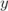direction. The result is that the normal gradient of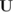is small along a plane coinciding with our boundary. We therefore impose the condition that the normal component is zero, i.e. specify the boundary as a symmetryPlane, thereby ensuring that the comparison with the analytical is reasonable.

### 2.2.5 Running the case

No fluid properties need be specified in this problem since the flow is assumed to be incompressible and inviscid. In the system subdirectory, the controlDict specifies the control parameters for the run. Note that since we assume steady flow, we only run for 1 time step:

1/*--------------------------------*- C++ -*----------------------------------*\
2| =========                 |                                                 |
3| \\      /  F ield         | OpenFOAM: The Open Source CFD Toolbox           |
4|  \\    /   O peration     | Version:  v2006                                 |
5|   \\  /    A nd           | Website:  www.openfoam.com                      |
6|    \\/     M anipulation  |                                                 |
7\*---------------------------------------------------------------------------*/
8FoamFile
9{
10    version     2.0;
11    format      ascii;
12    class       dictionary;
13    location    "system";
14    object      controlDict;
15}
16// * * * * * * * * * * * * * * * * * * * * * * * * * * * * * * * * * * * * * //
17
18application     potentialFoam;
19
20startFrom        latestTime;
21
22startTime       0;
23
24stopAt           nextWrite;
25
26endTime         1;
27
28deltaT          1;
29
30writeControl     timeStep;
31
32writeInterval    1;
33
34purgeWrite      0;
35
36writeFormat     ascii;
37
38writePrecision  6;
39
40writeCompression off;
41
42timeFormat      general;
43
44timePrecision   6;
45
46runTimeModifiable true;
47
48functions
49{
50    error
51    {
52        name    error;
53        type    coded;
54        libs    (utilityFunctionObjects);
55
56        codeEnd
57        #{
58            // Lookup U
59            Info<< "Looking up field U\n" << endl;
60            const volVectorField& U = mesh().lookupObject<volVectorField>("U");
61
62            Info<< "Reading inlet velocity uInfX\n" << endl;
63
64            scalar ULeft = 0.0;
65            label leftI = mesh().boundaryMesh().findPatchID("left");
66            const fvPatchVectorField& fvp = U.boundaryField()[leftI];
67            if (fvp.size())
68            {
69                ULeft = fvp.x();
70            }
71            reduce(ULeft, maxOp<scalar>());
72
73            dimensionedScalar uInfX("uInfx", dimVelocity, ULeft);
74
75            Info<< "U at inlet = " << uInfX.value() << " m/s" << endl;
76
77
78            scalar magCylinder = 0.0;
79            label cylI = mesh().boundaryMesh().findPatchID("cylinder");
80            const fvPatchVectorField& cylFvp = mesh().C().boundaryField()[cylI];
81            if (cylFvp.size())
82            {
83                magCylinder = mag(cylFvp);
84            }
85            reduce(magCylinder, maxOp<scalar>());
86
88
89            Info<< "Cylinder radius = " << radius.value() << " m" << endl;
90
91            volVectorField UA
92            (
93                IOobject
94                (
95                    "UA",
96                    mesh().time().timeName(),
97                    U.mesh(),
99                    IOobject::AUTO_WRITE
100                ),
101                U
102            );
103
104            Info<< "\nEvaluating analytical solution" << endl;
105
106            const volVectorField& centres = UA.mesh().C();
107            volScalarField magCentres(mag(centres));
108            volScalarField theta(acos((centres & vector(1,0,0))/magCentres));
109
110            volVectorField cs2theta
111            (
112                cos(2*theta)*vector(1,0,0)
113              + sin(2*theta)*vector(0,1,0)
114            );
115
116            UA = uInfX*(dimensionedVector(vector(1,0,0))
118
119            // Force writing of UA (since time has not changed)
120            UA.write();
121
122            volScalarField error("error", mag(U-UA)/mag(UA));
123
124            Info<<"Writing relative error in U to " << error.objectPath()
125                << endl;
126
127            error.write();
128        #};
129    }
130}
131
132
133// ************************************************************************* //

potentialFoam executes an iterative loop around the pressure equation which it solves in order that explicit terms relating to non-orthogonal correction in the Laplacian term may be updated in successive iterations. The number of iterations around the pressure equation is controlled by the nNonOrthogonalCorrectors keyword in the fvSolution dictionary. In the first instance we can set nNonOrthogonalCorrectors to 0 so that no loops are performed, i.e. the pressure equation is solved once, and there is no non-orthogonal correction. The solution is shown in Figure 2.17(a) (at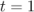, when the steady-state simulation is complete).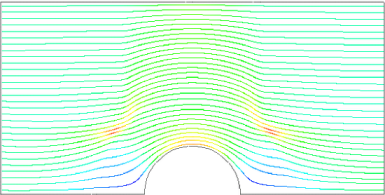(a) With no non-orthogonal correction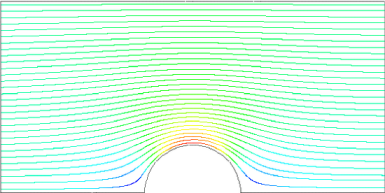(b) With non-orthogonal correction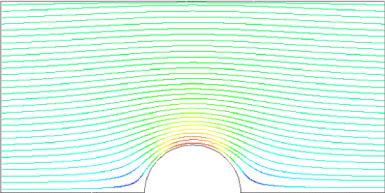(c) Analytical solution

Figure 2.17: Streamlines of potential flow

We expect the solution to show smooth streamlines passing across the domain as in the analytical solution in Figure 2.17(c), yet there is clearly some error in the regions where there is high non-orthogonality in the mesh, e.g. at the join of blocks 0, 1 and 3. The case can be run a second time with some non-orthogonal correction by setting nNonOrthogonalCorrectors to 3. The solution shows smooth streamlines with no significant error due to non-orthogonality as shown in Figure 2.17(b).